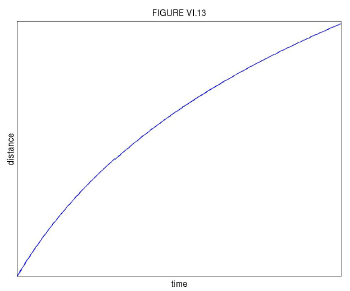$$\require{cancel}$$

# 6.4.4A: Resistive Force Only

•• Contributed by Jeremy Tatum
• Emeritus Professor (Physics & Astronomy) at University of Victoria

We'll imagine a puck sliding along a frictionless surface against turbulent air resistance. The Equation of motion is:

$\ddot{x}=-kv^{2}.\label{eq:6.4.1}$

By this time we assume that the student knows how to obtain the first and second time integrals and the space integral. The actual integrations may be slightly more difficult, but we leave it to the reader to obtain the results

$v=\frac{v_{0}}{1+kv_{0}t}$

$x=\frac{\ln(1+kv_{0}t)}{k}$

$v=v_{0}e^{-kx}.$

These are illustrated in Figures VI.12,13,14. Note that, provided that Equation $$\ref{eq:6.4.1}$$ accurately describes the entire motion (which may not be the case in a practical situation), there is no finite limit to x, nor does the speed drop to zero in any finite time.